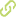# Propagation dynamics of vector Mathieu-Gauss beams•
• Overview
•
• Identity
•
•
• View All
•

### Abstract

• The vector Mathieu-Gauss beams of integer order are examined as the solutions of the vector paraxial wave equation in elliptical coordinates. The propagation of the vector components and the three-dimensional intensity distribution of focused vector Mathieu-Gauss beams are analyzed for a variety of polarizations. Conditions in which the linearly polarized Mathieu-Gauss beams can be approximated by the scalar solutions of the paraxial wave equation are also discussed.

### Publication date

• November 6, 2006